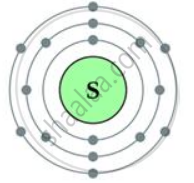# Sulphur Has an Atomic Number 16 and a Mass of 32. State the Number of Protons and Neutrons in the Nucleus of Sulphur. Give a Simple Diagram to Show the Arrangement of Electrons in an Atom of Sulphur. - Chemistry

Short Note

Sulphur has an atomic number 16 and a mass of 32.

State the number of protons and neutrons in the nucleus of sulphur. Give a simple diagram to show the arrangement of electrons in an atom of sulphur.

#### Solution

Atomic number = 16

Atomic mass = 32

Number of protons = 16

Number of electrons = 16

Number of neutrons = 32 - 16 = 16

Electronic configuration = 2, 8, 6Concept: Atomic Number (Z)
Is there an error in this question or solution?

#### APPEARS IN

Selina Concise Science Chemistry 1 Class 9 ICSE
Chapter 4 Atomic Structure and Chemical Bonding
Exercise 4 (B) | Q 7 | Page 63